Multitrait-Multimethod Matrix

# The Multitrait-Multimethod Matrix

## What is the Multitrait-Multimethod Matrix?

The Multitrait-Multimethod Matrix (hereafter labeled MTMM) is an approach to assessing the construct validity of a set of measures in a study. It was developed in 1959 by Campbell and Fiske (Campbell, D. and Fiske, D. (1959). Convergent and discriminant validation by the multitrait-multimethod matrix. 56, 2, 81-105.) in part as an attempt to provide a practical methodology that researchers could actually use (as opposed to the nomological network idea which was theoretically useful but did not include a methodology). Along with the MTMM, Campbell and Fiske introduced two new types of validity – convergent and discriminant – as subcategories of construct validity. Convergent validity is the degree to which concepts that should be related theoretically are interrelated in reality. Discriminant validity is the degree to which concepts that should not be related theoretically are, in fact, not interrelated in reality. You can assess both convergent and discriminant validity using the MTMM. In order to be able to claim that your measures have construct validity, you have to demonstrate both convergence and discrimination.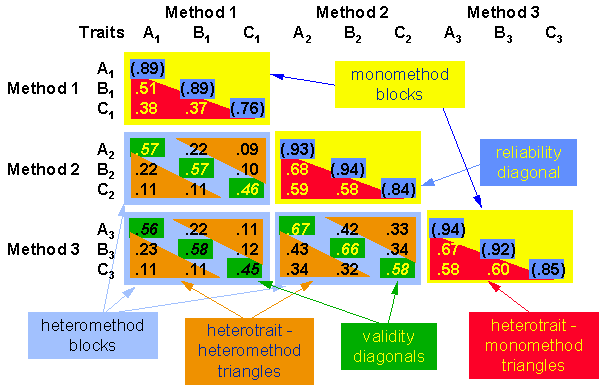The MTMM is simply a matrix or table of correlations arranged to facilitate the interpretation of the assessment of construct validity. The MTMM assumes that you measure each of several concepts (called traits by Campbell and Fiske) by each of several methods (e.g., a paper-and-pencil test, a direct observation, a performance measure). The MTMM is a very restrictive methodology – ideally you should measure each concept by each method.

To construct an MTMM, you need to arrange the correlation matrix by concepts within methods. The figure shows an MTMM for three concepts (traits A, B and C) each of which is measured with three different methods (1, 2 and 3) Note that you lay the matrix out in blocks by method. Essentially, the MTMM is just a correlation matrix between your measures, with one exception – instead of 1’s along the diagonal (as in the typical correlation matrix) we substitute an estimate of the reliability of each measure as the diagonal.

Before you can interpret an MTMM, you have to understand how to identify the different parts of the matrix. First, you should note that the matrix is consists of nothing but correlations. It is a square, symmetric matrix, so we only need to look at half of it (the figure shows the lower triangle). Second, these correlations can be grouped into three kinds of shapes: diagonals, triangles, and blocks. The specific shapes are:

### The Reliability Diagonal (monotrait-monomethod)

Estimates of the reliability of each measure in the matrix. You can estimate reliabilities a number of different ways (e.g., test-retest, internal consistency). There are as many correlations in the reliability diagonal as there are measures – in this example there are nine measures and nine reliabilities. The first reliability in the example is the correlation of Trait A, Method 1 with Trait A, Method 1 (hereafter, I’ll abbreviate this relationship A1-A1). Notice that this is essentially the correlation of the measure with itself. In fact such a correlation would always be perfect (i.e., r=1.0). Instead, we substitute an estimate of reliability. You could also consider these values to be monotrait-monomethod correlations.

### The Validity Diagonals (monotrait-heteromethod)

Correlations between measures of the same trait measured using different methods. Since the MTMM is organized into method blocks, there is one validity diagonal in each method block. For example, look at the A1-A2 correlation of .57. This is the correlation between two measures of the same trait (A) measured with two different measures (1 and 2). Because the two measures are of the same trait or concept, we would expect them to be strongly correlated. You could also consider these values to be monotrait-heteromethod correlations.

### The Heterotrait-Monomethod Triangles

These are the correlations among measures that share the same method of measurement. For instance, A1-B1 = .51 in the upper left heterotrait-monomethod triangle. Note that what these correlations share is method, not trait or concept. If these correlations are high, it is because measuring different things with the same method results in correlated measures. Or, in more straightforward terms, you’ve got a strong “methods” factor.

### Heterotrait-Heteromethod Triangles

These are correlations that differ in both trait and method. For instance, A1-B2 is .22 in the example. Generally, because these correlations share neither trait nor method we expect them to be the lowest in the matrix.

### The Monomethod Blocks

These consist of all of the correlations that share the same method of measurement. There are as many blocks as there are methods of measurement.

### The Heteromethod Blocks

These consist of all correlations that do not share the same methods. There are (K(K-1))/2 such blocks, where K = the number of methods. In the example, there are 3 methods and so there are (3(3-1))/2 = (3(2))/2 = 62 = 3 such blocks.

## Principles of Interpretation

Now that you can identify the different parts of the MTMM, you can begin to understand the rules for interpreting it. You should realize that MTMM interpretation requires the researcher to use judgment. Even though some of the principles may be violated in an MTMM, you may still wind up concluding that you have fairly strong construct validity. In other words, you won’t necessarily get perfect adherence to these principles in applied research settings, even when you do have evidence to support construct validity. To me, interpreting an MTMM is a lot like a physician’s reading of an x-ray. A practiced eye can often spot things that the neophyte misses! A researcher who is experienced with MTMM can use it to identify weaknesses in measurement as well as for assessing construct validity.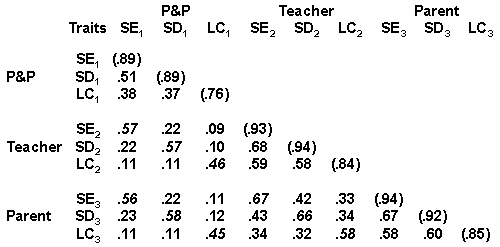To help make the principles more concrete, let’s make the example a bit more realistic. We’ll imagine that we are going to conduct a study of sixth grade students and that we want to measure three traits or concepts: Self Esteem (SE), Self Disclosure (SD) and Locus of Control (LC). Furthermore, let’s measure each of these three different ways: a Paper-and-Pencil (P&P) measure, a Teacher rating, and a Parent rating. The results are arrayed in the MTMM. As the principles are presented, try to identify the appropriate coefficients in the MTMM and make a judgement yourself about the strength of construct validity claims.

The basic principles or rules for the MTMM are:

Coefficients in the reliability diagonal should consistently be the highest in the matrix. That is, a trait should be more highly correlated with itself than with anything else! This is uniformly true in our example.

Coefficients in the validity diagonals should be significantly different from zero and high enough to warrant further investigation. This is essentially evidence of convergent validity. All of the correlations in our example meet this criterion.

A validity coefficient should be higher than values lying in its column and row in the same heteromethod block. In other words, (SE P&P)-(SE Teacher) should be greater than (SE P&P)-(SD Teacher), (SE P&P)-(LC Teacher), (SE Teacher)-(SD P&P) and (SE Teacher)-(LC P&P). This is true in all cases in our example.

A validity coefficient should be higher than all coefficients in the heterotrait-monomethod triangles. This essentially emphasizes that trait factors should be stronger than methods factors. Note that this is not true in all cases in our example. For instance, the (LC P&P)-(LC Teacher) correlation of .46 is less than (SE Teacher)-(SD Teacher), (SE Teacher)-(LC Teacher), and (SD Teacher)-(LC Teacher) – evidence that there might me a methods factor, especially on the Teacher observation method.

The same pattern of trait interrelationship should be seen in all triangles. The example clearly meets this criterion. Notice that in all triangles the SE-SD relationship is approximately twice as large as the relationships that involve LC.

The MTMM idea provided an operational methodology for assessing construct validity. In the one matrix it was possible to examine both convergent and discriminant validity simultaneously. By its inclusion of methods on an equal footing with traits, Campbell and Fiske stressed the importance of looking for the effects of how we measure in addition to what we measure. And, MTMM provided a rigorous framework for assessing construct validity.

Despite these advantages, MTMM has received little use since its introduction in 1959. There are several reasons. First, in its purest form, MTMM requires that you have a fully-crossed measurement design – each of several traits is measured by each of several methods. While Campbell and Fiske explicitly recognized that one could have an incomplete design, they stressed the importance of multiple replication of the same trait across method. In some applied research contexts, it just isn’t possible to measure all traits with all desired methods (would you use an “observation” of weight?). In most applied social research, it just wasn’t feasible to make methods an explicit part of the research design. Second, the judgmental nature of the MTMM may have worked against its wider adoption (although it should actually be perceived as a strength). many researchers wanted a test for construct validity that would result in a single statistical coefficient that could be tested – the equivalent of a reliability coefficient. It was impossible with MTMM to quantify the degree of construct validity in a study. Finally, the judgmental nature of MTMM meant that different researchers could legitimately arrive at different conclusions.

## A Modified MTMM – Leaving out the Methods Factor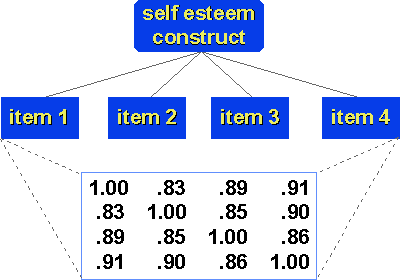As mentioned above, one of the most difficult aspects of MTMM from an implementation point of view is that it required a design that included all combinations of both traits and methods. But the ideas of convergent and discriminant validity do not require the methods factor. To see this, we have to reconsider what Campbell and Fiske meant by convergent and discriminant validity.

## What is convergent validity?

It is the principle that measures of theoretically similar constructs should be highly intercorrelated. We can extend this idea further by thinking of a measure that has multiple items, for instance, a four-item scale designed to measure self-esteem. If each of the items actually does reflect the construct of self-esteem, then we would expect the items to be highly intercorrelated as shown in the figure. These strong intercorrelations are evidence in support of convergent validity.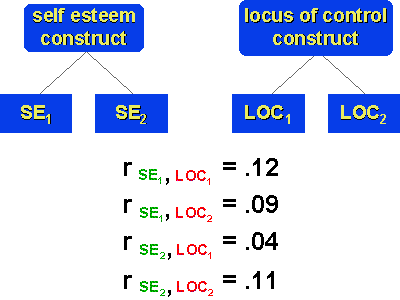## And what is discriminant validity?

It is the principle that measures of theoretically different constructs should not correlate highly with each other. We can see that in the example that shows two constructs – self-esteem and locus of control – each measured in two instruments. We would expect that, because these are measures of different constructs, the cross-construct correlations would be low, as shown in the figure. These low correlations are evidence for validity. Finally, we can put this all together to see how we can address both convergent and discriminant validity simultaneously. Here, we have two constructs – self-esteem and locus of control – each measured with three instruments. The red and green correlations are within-construct ones. They are a reflection of convergent validity and should be strong. The blue correlations are cross-construct and reflect discriminant validity. They should be uniformly lower than the convergent coefficients.

The important thing to notice about this matrix is that it does not explicitly include a methods factor as a true MTMM would. The matrix examines both convergent and discriminant validity (like the MTMM) but it only explicitly looks at construct intra- and interrelationships. We can see in this example that the MTMM idea really had two major themes. The first was the idea of looking simultaneously at the pattern of convergence and discrimination. This idea is similar in purpose to the notions implicit in the nomological network – we are looking at the pattern of interrelationships based upon our theory of the nomological net. The second idea in MTMM was the emphasis on methods as a potential confounding factor.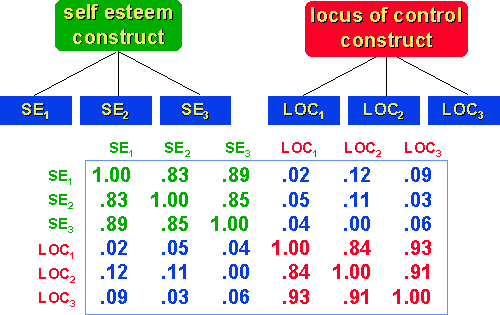While methods may confound the results, they won’t necessarily do so in any given study. And, while we need to examine our results for the potential for methods factors, it may be that combining this desire to assess the confound with the need to assess construct validity is more than one methodology can feasibly handle. Perhaps if we split the two agendas, we will find that the possibility that we can examine convergent and discriminant validity is greater. But what do we do about methods factors? One way to deal with them is through replication of research projects, rather than trying to incorporate a methods test into a single research study. Thus, if we find a particular outcome in a study using several measures, we might see if that same outcome is obtained when we replicate the study using different measures and methods of measurement for the same constructs. The methods issue is considered more as an issue of generalizability (across measurement methods) rather than one of construct validity.

When viewed this way, we have moved from the idea of a MTMM to that of the multitrait matrix that enables us to examine convergent and discriminant validity, and hence construct validity. We will see that when we move away from the explicit consideration of methods and when we begin to see convergence and discrimination as differences of degree, we essentially have the foundation for the pattern matching approach to assessing construct validity.